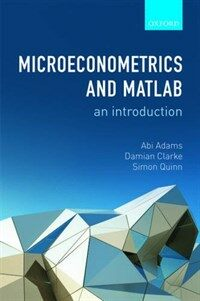> 상세정보

상세정보Microeconometrics and MATLAB : an introduction (9회 대출)

자료유형
단행본
개인저자
Adams, Abi. Clarke, Damian. Quinn, Simon, 1981-.
서명 / 저자사항
Microeconometrics and MATLAB : an introduction / Abi Adams, Damian Clarke, Simon Quinn.
발행사항
Oxford :   Oxford University Press,   c2015.
형태사항
xvii, 195 p. : ill. ; 24 cm.
ISBN
9780198754497 (hbk.) 9780198754503 (pbk.)
서지주기
Includes bibliographical references and index.
일반주제명
Econometrics. Economics --Mathematical models. Numerical analysis --Computer programs.
 000 00000cam u2200205 a 4500 001 000045871387 005 20160510095332 008 160509s2015 enka b 001 0 eng d 015 ▼a GBB5B9272 ▼2 bnb 020 ▼a 9780198754497 (hbk.) 020 ▼a 9780198754503 (pbk.) 035 ▼a (KERIS)REF000018007457 040 ▼a EQO ▼b eng ▼e rda ▼c EQO ▼d 211009 082 0 4 ▼a 330.015195 ▼2 23 084 ▼a 330.015195 ▼2 DDCK 090 ▼a 330.015195 ▼b A211m 100 1 ▼a Adams, Abi. 245 1 0 ▼a Microeconometrics and MATLAB : ▼b an introduction / ▼c Abi Adams, Damian Clarke, Simon Quinn. 260 ▼a Oxford : ▼b Oxford University Press, ▼c c2015. 300 ▼a xvii, 195 p. : ▼b ill. ; ▼c 24 cm. 504 ▼a Includes bibliographical references and index. 630 0 0 ▼a MATLAB. 650 0 ▼a Econometrics. 650 0 ▼a Economics ▼x Mathematical models. 650 0 ▼a Numerical analysis ▼x Computer programs. 700 1 ▼a Clarke, Damian. 700 1 ▼a Quinn, Simon, ▼d 1981-. 945 ▼a KLPA

소장정보

No. 소장처 청구기호 등록번호 도서상태 반납예정일 예약 서비스
No. 1 소장처 청구기호 330.015195 A211m 등록번호 121236426 도서상태 대출가능 반납예정일 예약 서비스

컨텐츠정보

목차

CONTENTS
LIST OF FIGURES = ix
LIST OF TABLES = xi
PROLOGUE = xiii
INTRODUCTION = xv
PART Ⅰ FOUNDATIONS = 1
1 Entering the 'Matrix Laboratory' = 3
1.1 OLS in MATLAB : 'Hello, world!' = 5
1.2 The Beauty of Functions = 8
1.3 A Simple Utility Function = 13
1.4 Review and Exercises = 17
2 The Agent Optimizes = 20
2.1 Profit Maximization = 20
2.2 Utility Maximization = 23
2.3 Simulating Economic Models = 27
2.4 Review and Exercises = 30
3 The Economist Optimizes = 31
3.1 Maximum Likelihood = 31
3.2 Generalized Method of Moments = 34
3.3 Review and Exercises = 38
PART Ⅱ DISCRETE CHOICE = 39
4 Discrete Multinomial Choice = 41
4.1 Binary Logit = 42
4.2 Multinomial Logit = 48
4.3 Multinomial Probit = 52
4.4 Review and Exercises = 60
5 Discrete Games = 62
5.1 A Simple Cournot Game = 62
5.2 A Discrete Bayesian Game = 69
5.3 Review and Exercises = 79
PART Ⅲ DYNAMICS = 81
6 Dynamic Choice on a Finite Horizon = 83
6.1 Direct Attack = 83
6.2 Dynamic Programming = 90
6.3 Memoization = 94
6.4 Stochastic Dynamic Programming = 95
6.5 Estimating Finite Horizon Models = 99
6.6 Review and Exercises = 102
7 Dynamic Choice on an Infinite Horizon = 105
7.1 Value Function Iteration = 106
7.2 Policy Function Iteration = 113
7.3 Estimating Infinite Horizon Models = 116
7.4 Review and Exercises = 117
PART Ⅳ NONPARAMETRIC METHODS = 123
8 Nonparametric Regression = 125
8.1 Parametric Versus Nonparametric Approaches = 125
8.2 Kernel Regression = 128
8.3 Cross Validation = 140
8.4 Local Linear Regression = 145
8.5 Review and Exercises = 147
9 Semiparametric Methods = 150
9.1 Multivariate Kernel Regression = 151
9.2 Dimension Reduction = 155
9.3 Partially Linear Models = 156
9.4 Single Index Models = 160
9.5 Review and Exercises = 166
PART Ⅴ SPEED = 169
10 Speeding Things Up... = 171
10.1 Introduction = 171
10.2 Clever Coding = 171
10.3 Parallel Computing = 179
10.4 Parallel Computing with the GPU = 181
10.5 Other Tricks = 185
11 ...and Slowing Things Down = 186
BIBLIOGRAPHY = 189
INDEX = 193

관련분야 신착자료

남준우 (2021)

김동하 (2021)

Nudge : the final edition

Thaler, Richard H. (2021)

The China paradox : at the front line of economic transformation / 2nd ed

Clifford, Paul Gilmore (2022)

(본질적인) 제임스 뷰캐넌

Boudreaux, Donald J (2021)

김인규 (2021)

찰리 멍거의 말들 : 투자, 경제, 비즈니스 그리고 삶에 관하여

Munger, Charles T. (2021)

김일태 (2021)

성태경 (2022)

(맨큐의) 핵심경제학 / 제9판

Mankiw, N. Gregory (2021)

강문성 (2021)

이한식 (2021)

Markets in the making : rethinking competition, goods, and innovation

Callon, Michel (2021)

The power of creative destruction : economic upheaval and the wealth of nations

Aghion, Philippe (2021)

최배근 (2021)

Cogs and monsters : what economics is, and what it should be

Coyle, Diane (2021)

An economist's lessons on happiness : farewell dismal science!

Easterlin, Richard A. (2021)